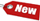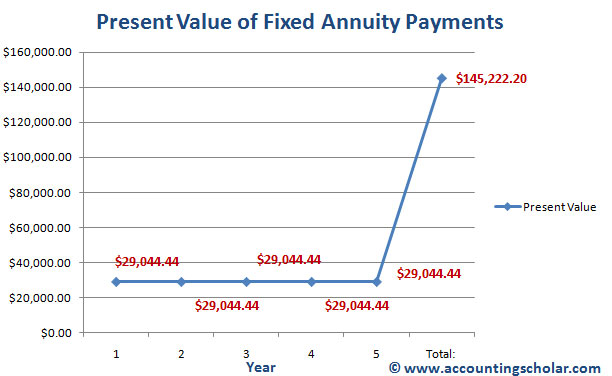Browse Accounting Lessons HereAccounting Terms & DefinitionsAccounting for Merchandising ActivitiesDebits and Credits (Double Entry Accounting)Business Valuation FormulasTime Value of Money & Present/Future ValuesComplex Debt & Equity InstrumentsCommon Stock & Shareholder's EquityAccounting & Finance RatiosValuing Common StockCorporate Income TaxesLower of Cost or Market (LCM) & Inventory ValuationChart of Accounts & BookkeepingBonds Payable & Long Term LiabilitiesCapital AssetsGAAP, Accrual & Cash Accounting, Information Commodity, Internal Controls & MaterialityWhat category of browser are you on this website? Accounting student (homework help) Finance professor (university research) Accounting manager (at work) Other Explore Careers in Accounting and FinanceVisit our section on Careers in Accounting & Finance to explore vast opportunities in this industry.

Chapter 4.16® - Calculating Annuity Payments using Annuity Present Value Factor – Example

Just like how we calculated the present value of future annuity payments now, we can calculate the vice versa meaning we can calculate the future value of cash flow payments (PMT) given a present value today. Remember in these calculations, we do not need the Future value (FV) information. Let’s take an example; suppose you want to start up a new business and need \$100,000 to start up, market and run your business. Because you feel the business will be short lived for some reason, you would like to pay off this \$100,000 balance in a set of five equal payments. Given the interest rate is 14%, what will your monthly annuity payments (five of them) be?

Notice in this example we know the present value is \$100,000 and the interest rate on the loan is 14%. Since these payments are spread over 5 periods and the payments will be equal, what will the payments be? We will use the present value of an annuity formula to derive our answer:

 Annuity Present Value = C x [(1 – Present Value Factor) / r] \$100,000 = C x [(1 – \$1/ (1 + r)t) / r] \$100,000 = C x [(1 – \$1/ (1 + 0.14)5) / 0.14] \$100,000 = C x [(1 – \$1/ (1.93) / 0.14] \$100,000 = C x [(1 – 0.518) / 0.14] \$100,000 = C x [(0.482) / 0.14] \$100,000 = C x 3.443 C = \$100,000 / 3.443 C = \$29,044.44

This therefore means you will have to make annuity payments of \$29,044.44 every year for the next 5 years to pay off the \$100,000 loan at 14% interest rate.

Here’s how to do this exact calculation using our BAII plus financial calculator:

Find annuity PMTs of \$100,000 PV at Year 5, 14% borrowing rate using our Financial Calculator

 N = 5 I/Y = 14% PV = \$-100,000 PMT = ? FV = \$0 2ND I/Y P/Y = 1 C/Y = 1 CPT & PV = \$29,128.35

Below is a graphical representation of this data.This graph above shows the equal annual payments of \$29,044.44 that will pay off the entire loan in 5 years at 14% interest rate. The graph also indicates to us that the total cost of the loan would be \$145,222.20 which indicates total interest will be \$145,222.20 - \$100,000 = \$45,222.20